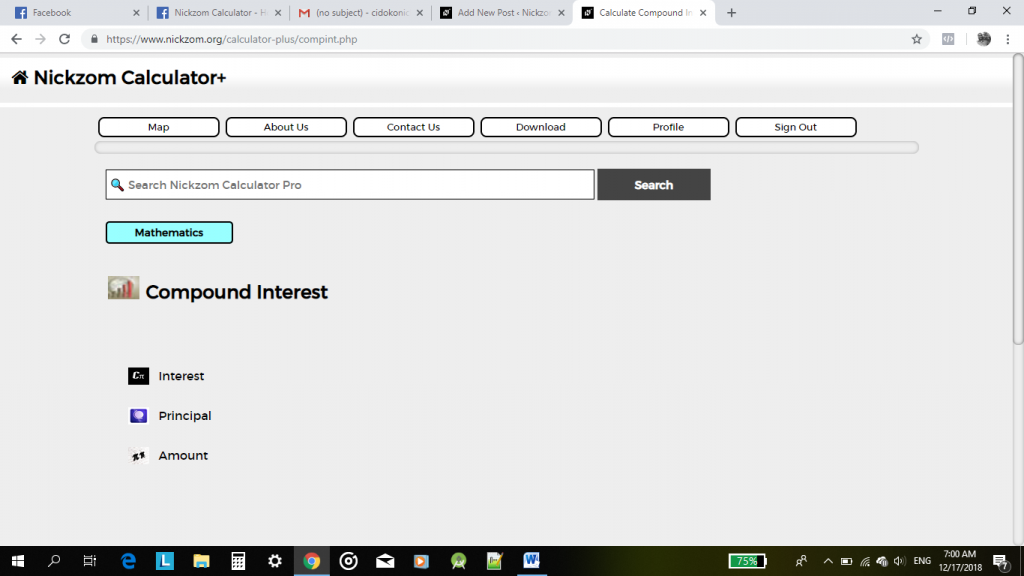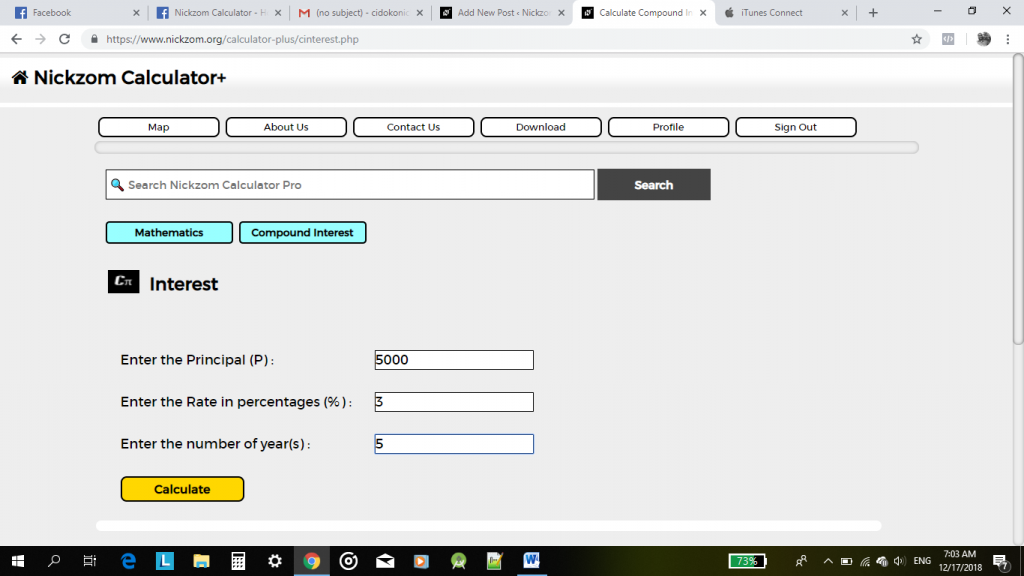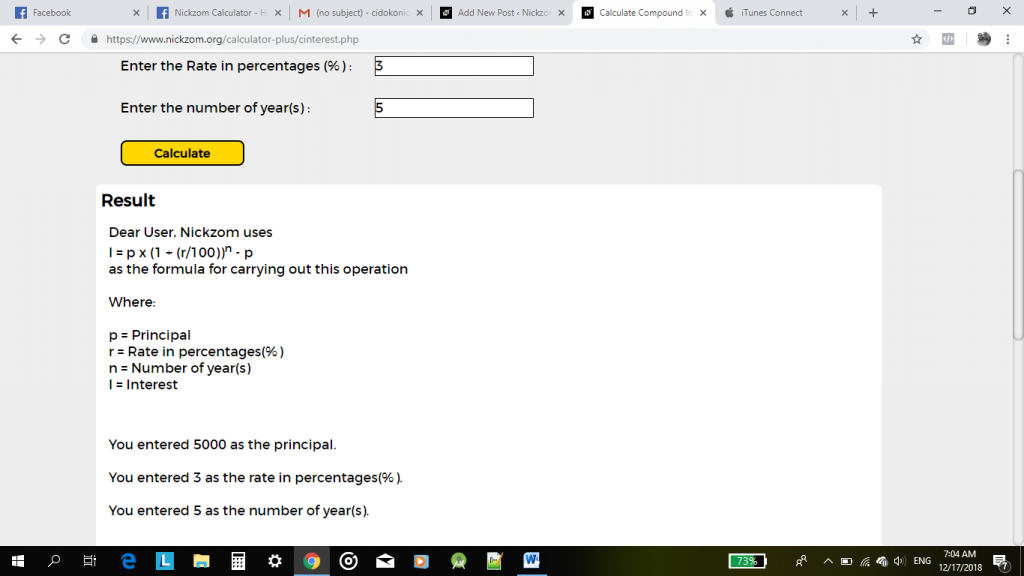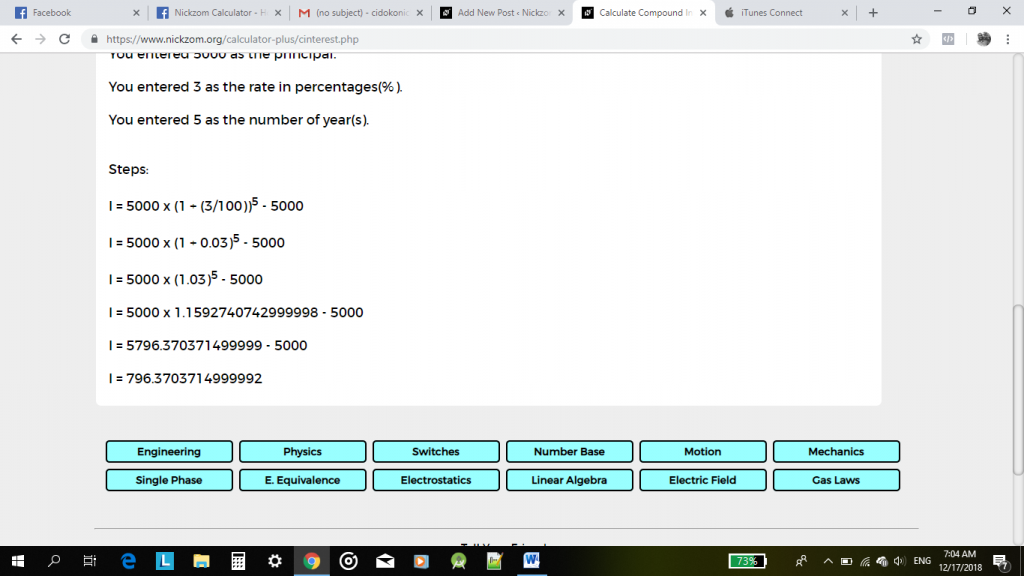# Calculating Compound Interest Using Nickzom Calculator

Using the Nickzom Calculator for Math calculations is quite simple. Navigation through any of the App platforms from Mobile to Web is quite easy. Just a bit of playing around and you’re quite comfortable with the user interface. Does Math seem hard, Nickzom Calculator makes it easier.

We will consider Compound Interest, how your Principal, Interest and Amount can easily be calculated using the Nickzom calculator. The platform  also goes a long way in showing the formula used, and the steps it took to arrive at its answer.Compound Interest

Compound Interest being a very popular topic in Mathematics entails basically  Principal, Rate, Interest, Time (number of years) and expected amount. The Nickzom Calculator can carry out calculations on Compound Interest, solving problems on

• Interest (I)
• Principal (P)
• Amount (A)

To calculate Compound Interest problems on Nickzom Calculator, simply open the Nickzom platform you have and select the Subject of interest, which is Mathematics in this case. Search through all the topics available under the Math section to find “Compound Interest”.

Under the Compound Interest section you will find 3 subsections on Interest, Principal and Amount. Depending on what you wish to solve you can select any of the three.

To calculate for your Interest, you need to have three variables available; your Principal (P), Rate in percentage(%) and Time in years(s). Simply click on Interest and the platform will open, showing you various sections of Principal, Rate and Time which you will be required to fill. Once these sections are filled, click on the calculate interface and Nickzom shows you the formula used, what each Variable symbol represent and the sequential steps that led to the answer.

To calculate for Amount simply click on Amount, fill in your PRT (Principal, Rate and Time), and click calculate and your answer comes out with the formula used what each Variable symbol represent and the procedures.

To calculate for Principal, the same procedures above applies. Click on Principal and the platform opens. The only difference is rather than the PRT (Principal, Rate and Time), you will have to input your Amount in place for Principal, quite logical right, it’s actually the amount we are looking for. Once you’re done filling the required sections, click calculate and the Formula, Variable symbol representation, procedures and answer will be displayed.

Calculating Compound Interest has never been easier, and this has been made possible by the Nickzom calculator. You can do so much more with the Pro version. Try it, download it and don’t forget to rate it.Nickzom Calculator (The Calculator Encyclopedia) is able and capable of providing us with this information on a platter. You can access Nickzom Calculator via any of these means:
Web – https://www.nickzom.org/calculator-plus